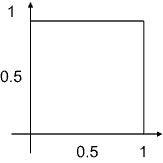# How To: Work with Geometric Model

We manipulate with the geometric model and the finite element mesh using a Model object. The Model object represents the geometric model document. To create a new Model object we can use the Add method of the corresponding document collection.

Dim QF as QuickField.Application
......
Dim mdl as QuickField.Model

Another way (and probably the most straightforward) way to get a Model object is the Model property of the Problem object. This can be obtained by the Problem it is referred to or by the collection of the model. In the following example, we get an empty Model object from the newly created problem:

Dim prbNew As QuickField.Problem
Dim mdl As QuickField.Model

Set mdl = prbNew.Model

Opening an existing model is done in a similar manner. We can do it by Open method of the document collection or using the Model property of the Problem object.

## Geometric Entities and Collections

In dealing with a geometric model, we operate with the following entities: vertices, edges and blocks. There is a separate object for each kind of geometric entity (Vertex, Edge and Block respectively). These three inherit their methods and properties from a more basic Shape object. In some situations it is more convenient to manipulate with Shape object without regards to its details.

There are two different kinds of collection of a Shape objects. One of them is a Shapes collection. There is only one Shapes collection for each model and it contains all the Shapes (Vertices, Blocks and Edges) in a model. It is accessible by the Shapes property of the Model object. The Shapes collection provides methods for creating a new Vertex and Edge. For example, the following example creates a square with a lower left corner at (0, 0) and upper right corner at (1, 1):

 Dim prb as QuickField.Problem     ......... Dim mdl As QuickField.Model Set mdl = prb.Model With mdl.Shapes     .AddEdge QF.PointXY(0, 1), QF.PointXY(1, 1)     .AddEdge QF.PointXY(1, 1), QF.PointXY(1, 0)     .AddEdge QF.PointXY(1, 0), QF.PointXY(0, 0)     .AddEdge QF.PointXY(0, 0), QF.PointXY(0, 1) End WithAnother kind of collection of Shapes is a ShapeRange object. A ShapeRange is a collection including Shape objects that are temporary selected for some purpose. You can build as much ShapeRange collections as you need at the same time. Moreover, it is sometimes difficult to say in advance the nature of the object you are dealing with: a separate Shape or a ShapeRange. For example, the AddEdge of the Shapes collection returns the ShapeRange collection that contains the edge which just has been created. The collection might contain only one member as Edge, but there might be several adjacent edges if the new edge intersects one or more existing edges.

In general, you build your model by adding new vertices and edges to it in the some way you do it with the QuickField graphical user interface (GUI). As with creating new vertices and edges by GUI newly created objects might attract to the existing ones if the distance is less then the cell size of the grid. You control the cell size by the Grid object that you can get from the Model using Grid property.

## Creating Sub-Collections

The main difference between operating with your mouse in the QuickField window and defining the model programmatically is how to point the desired object (Vertex, Block or Edge). Here, with QuickField objects, you use often use some properties that return ShapeRange object, which is related to its parent Shape (or ShapeRange) in some way.

Among then are such properties as:

 Select shapes of the some type from the parent collection (only blocks, only edges or only vertices) Selects edges that build the boundary of the parent block(s). Selects shapes that are situated inside of given rectangle (circle). Returns a ShapeRange collection that contains the nearest block to the given point, the nearest edge and the nearest vertex (if any). Returns all the shapes labeled by the given label. These properties are applicable only for edges. Returns blocks that are adjacent to the edge from the left and the right side. You can assign an integer value to each shape (UserData) property. The Flagged property returns all the shapes assigned by the given value.